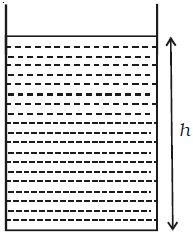# Pressure due to a Liquid Column

Let h be the height of the liquid column in a cylinder of cross sectional area A. If ρ is the density of the liquid, then weight of the liquid column W is given by

W = mass of liquid column x g = AhρgBy definition, pressure is the force acting per unit area.

So, Pressure = [weight of liquid column / (area of cross – section)]

= Ahρg/A = hρg

So, P = hρg